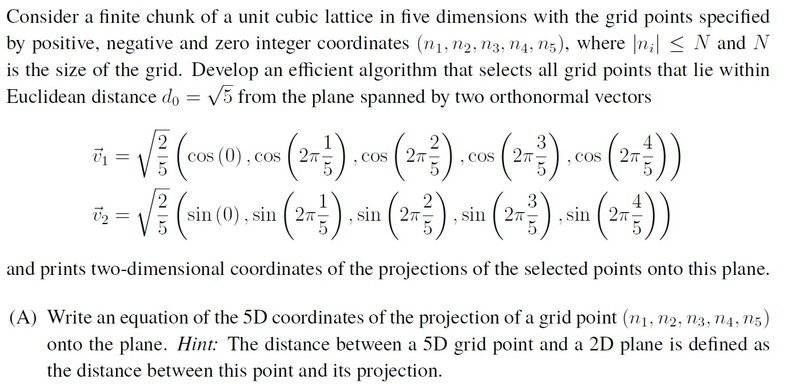# Equation of the 5D coordinates of the projection of a point

• user199

#### user199

Homework Statement
I have no idea how to calculate the equation of the 5D coordinates of the projection onto the plane as stated in the part A of the attached problem. Help in this regards would be greatly appreciated.
Relevant Equations
I only know the solution in 3D but in higher dimensions I have no idea how to calculate the equation of the coordinates of the projection onto the plane. My solution for 3D problem is:

A given point A(x0, y0, z0) and its projection A′ determine a line of which the direction vector s
coincides with the normal vector N of the projection plane P. As the point A′ lies at the same time on the line AA′ and the plane P, the coordinates of the radius (position) vector of a variable point of the line written in the parametric form
x = x0 + a · t,
y = y0 + b· t
and
z = z0+ c· t,
These variable coordinates of a point of the line plugged into the equation of the plane will determine the value of the parameter t such that this point will be, at the same time, on the line and the plane.

Example: Find the orthogonal projection of the point A(5, -6, 3) onto the plane 3x -2y + z -2 = 0.
Solution: The direction vector of the line AA′
is s = N = 3i -2 j + k, so the parametric equation of
the line which is perpendicular to the plane and passes through the given point A
these coordinates of the radius vector of the point A′ must satisfy the equation of the given plane that is
3 · (3t+ 5) -2 · (-2t -6)+ 1 · (t+ 3) -2 = 0 => t = -2
therefore, the coordinates of the point A′ are,
x =3t+ 5 =3 · (-2)+ 5 = -1, y = -2t-6 = -2· (-2)-6 = -2
and
z = t+ 3 = -2+ 3 = 1,
thus the orthogonal projection of the point A onto the given plane is A′(-1,-2,1).The hint implies to me finding the point in the plane nearest to the projected point.
Can you write a generic expression for a point in the plane?

The hint implies to me finding the point in the plane nearest to the projected point.
Can you write a generic expression for a point in the plane?